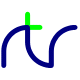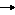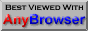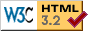## Appendix C - Binary and Hexadecimal

Numbers in Base 2 - Binary

Up to now, we have been content to think about computers putting numbers in little boxes called memory locations without being too concerned about how this actually happens. Largely, that's okay, like a car, you don't need to know how it works to get in it and drive to your destination. There comes a time however, when a little knowledge comes in useful and allows us to progress.

So, how does a computer store numbers? A computer's memory location is basically made up of switches. Millions of them. A switch can have two states: on or off. One switch on its own can therefore represent two numbers: 0 (off) and 1 (on). We combine groups of switches to allow us to store higher numbers. If we take two switches (call them 0 and 1) together, we can use this to represent 4 different numbers as there are four unique states. Here they are:

Switch Number
1 0
0 0 0
0 1 1
1 0 2
1 1 3

We go from right to left here, just as if we were reading 123 as being one hundred and twenty three. The lowest (right most) switch represents 1 when it's on. The next switch takes a value of 2 when switched on. To get the number represented, we add the values together. Let's add another switch:

Switch Number
2 1 0
0 0 0 0
0 0 1 1
0 1 0 2
0 1 1 3
1 0 0 4
1 0 1 5
1 1 0 6
1 1 1 7

Now we can represent numbers from 0 to 7. The first column assumes a value of 4 when on. We've got a pattern here, starting with switch 0:
 Switch 0 when on is worth 1 Switch 1 when on is worth 2 Switch 2 when on is worth 4
Each additional switch is worth twice the previous one. If we add another, its value will be 8 and allow us to represent numbers 0 to 15. This method of counting is termed binary (where 'bi' signifies two because we are dealing with two states). Each switch, mathematically, is 2 to the power of its position. Hence:
 2^0 = 1 2^1 = 2 2^2 = 4 2^3 = 8 etc.
When we talk about 8-bit numbers or 32-bit numbers, we're referring to the number of switches. Programmers call each switch a bit. Hence you can see that an 8-bit number is capable of representing 2^8 = 256 states. Here is what each bit is worth:

 2^7 2^6 2^5 2^4 2^3 2^2 2^1 2^0 128 64 32 16 8 4 2 1

Once you grasp this, 16 and 32 bit numbers are just the same, merely more bits. Sometimes the topmost bit is used to represent whether the number is negative, rather than giving it a numeric weight. Hence our 8-bit number above could be used to hold 0 to 255 or –128 to +127 depending on the convention used. 8-bits is such a common grouping that it has its own name: a byte. We have already seen that BB4W has a data type called a byte, so in this instance the top bit takes a numerical value rather than a sign and we can hold numbers 0 to 255.

Numbers in Base 16 - Hexadecimal

It is important to realize that representing something in binary is the same as representing something in decimal. The physical quantity doesn't change, just the means of representation. The method is similar to speaking a foreign language. We can say we have 'two cars' in English or 'deux voitures' in French. We can say 2 in decimal or 10 in binary, it's all the same quantity. Quantities can be represented in any number system that suits us. These systems are said to be in different bases. The base of a number is taken from the value of the second column of the representation.

 10 in decimal = ten hence we are in base 10 10 in binary = two hence we are in base 2
As humans, we can't work in binary for too long, all those 1's and 0's are just too confusing. What is needed is a way to represent a number which will quickly enable us to get back to binary should we need to. If we look at the pattern for the values of bits in binary (1,2,4,8,16 ...) we know that any of these numbers will use all the bits and so would be a good number base to use. If we take the next number after 2, we get 4. This is slightly better, but not much as our sequence of numbers now goes 0,1,2,3,10,11 etc. Try the next power of two, this is 8. This allows us to represent 0,1,2,3,4,5,6,7,10,11. That's getting there and base 8 numbers are used in some circles.

At the next number, 16, computer designers and engineers decided that this was the most convenient. Base 16 allows representation of numbers 0 to 15 (decimal) 0000 to 1111 (binary). Numbers in base 16 are called hexadecimal or hex for short. 10 in hex, 16 in decimal and 10000 in binary. As decimal runs out of characters at 9, the letters A to F are used to represent the missing numbers 10 to 15. BBC BASIC allows us to use hex numbers in our code. To let BASIC know the number is base 16, we put & in front of it. For the letters A to F use uppercase, it can be selected lowercase, but let's go with the flow here and use defaults. We can use binary numbers by prefixing them with % like this: %111, %1000. A complete list of all the numbers in 8 bits would be pretty boring to read in full so here are the edited highlights:

 Decimal Hexadecimal   Binary 0       &0       %00000000 1       &1       %00000001 2       &2       %00000010 3       &3       %00000011 4       &4       %00000100 5       &5       %00000101 6       &6       %00000110 7       &7       %00000111 8       &8       %00001000 9       &9       %00001001 10       &A       %00001010 11       &B       %00001011 12       &C       %00001100 13       &D       %00001101 14       &E       %00001110 15       &F       %00001111 16      &10       %00010000 17      &11       %00010001 18      &12       %00010010 19      &13       %00010011 ...      ...          ... 127      &7F       %01111111 128      &80       %10000000 129      &81       %10000001 ...      ...          ... 253      &FD       %11111101 254      &FE       %11111110 255      &FF       %11111111
Hex is so convenient because of this direct correlation to binary. One hex digit covers all possible combinations of four binary bits. The next digit just repeats the same pattern again.

Hex is so embedded in computers that BBC BASIC will even print it for us. In order to convert a number in decimal to a string representation in hex use STR\$ with tilde:

 PRINT STR\$~(255)
To convert a number back from hex to decimal use:
 PRINT EVAL ("&FFE")

### APPENDIX D